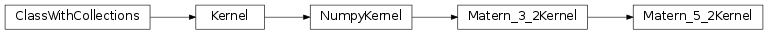# mvpa2.kernels.np.Matern_5_2Kernel¶class `mvpa2.kernels.np.``Matern_5_2Kernel`(**kwargs)

The Matern kernel class for the case ni=5/2.

This kernel is just Matern_3_2Kernel(numerator=5.0).

Attributes

 `descr` Description of the object if any

Methods

 `add_conversion`(typename, methodfull, methodraw) Adds methods to the Kernel class for new conversions `as_ls`(kernel) `as_np`() Converts this kernel to a Numpy-based representation `as_raw_ls`(kernel) `as_raw_np`() Directly return this kernel as a numpy array. `as_raw_sg`(kernel) Converts directly to a Shogun kernel `as_sg`(kernel) Converts this kernel to a Shogun-based representation `cleanup`() Wipe out internal representation `compute`(ds1[, ds2]) Generic computation of any kernel `computed`(\*args, \*\*kwargs) Compute kernel and return self `gradient`(data1, data2) Compute gradient of the kernel matrix. `reset`() `set_hyperparameters`(hyperparameter) Set hyperaparmeters from a vector.

Initialize a Squared Exponential kernel instance.

Parameters: length_scale : float or numpy.ndarray the characteristic length-scale (or length-scales) of the phenomenon under investigation. (Defaults to 1.0) enable_ca : None or list of str Names of the conditional attributes which should be enabled in addition to the default ones disable_ca : None or list of str Names of the conditional attributes which should be disabled sigma_f : float, optional Signal standard deviation. (Defaults to 1.0) numerator : float, optional the numerator of parameter ni of Matern covariance functions. Currently only numerator=3.0 and numerator=5.0 are implemented. (Defaults to 3.0)

Attributes

 `descr` Description of the object if any

Methods

 `add_conversion`(typename, methodfull, methodraw) Adds methods to the Kernel class for new conversions `as_ls`(kernel) `as_np`() Converts this kernel to a Numpy-based representation `as_raw_ls`(kernel) `as_raw_np`() Directly return this kernel as a numpy array. `as_raw_sg`(kernel) Converts directly to a Shogun kernel `as_sg`(kernel) Converts this kernel to a Shogun-based representation `cleanup`() Wipe out internal representation `compute`(ds1[, ds2]) Generic computation of any kernel `computed`(\*args, \*\*kwargs) Compute kernel and return self `gradient`(data1, data2) Compute gradient of the kernel matrix. `reset`() `set_hyperparameters`(hyperparameter) Set hyperaparmeters from a vector.